REFRESHER Linear Graphs INTERPRETING STRAIGHTLINE GRAPHS Equations and

• Slides: 21REFRESHER Linear GraphsINTERPRETING STRAIGHT-LINE GRAPHS Equations and graphs are used to study the relationship between two variables such as distance and speed. If a relationship exists between the variables, one can be said to be a function of the other. A function can be described by a table, a rule or a graph. We are going to analyze linear functions.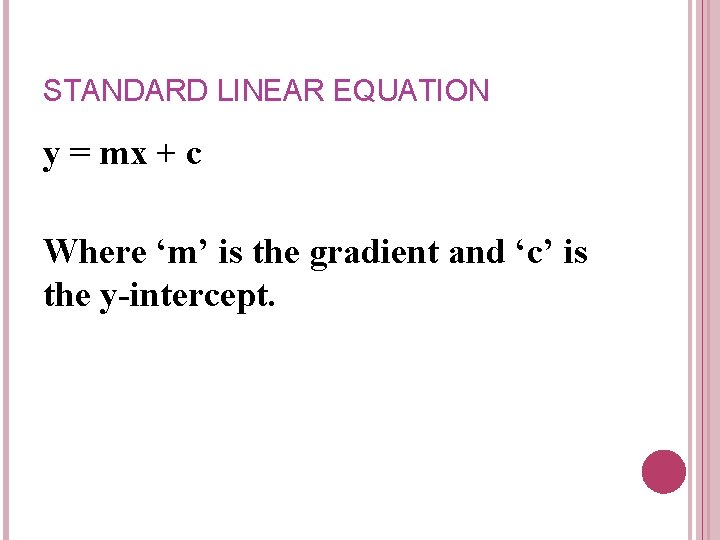STANDARD LINEAR EQUATION y = mx + c Where ‘m’ is the gradient and ‘c’ is the y-intercept.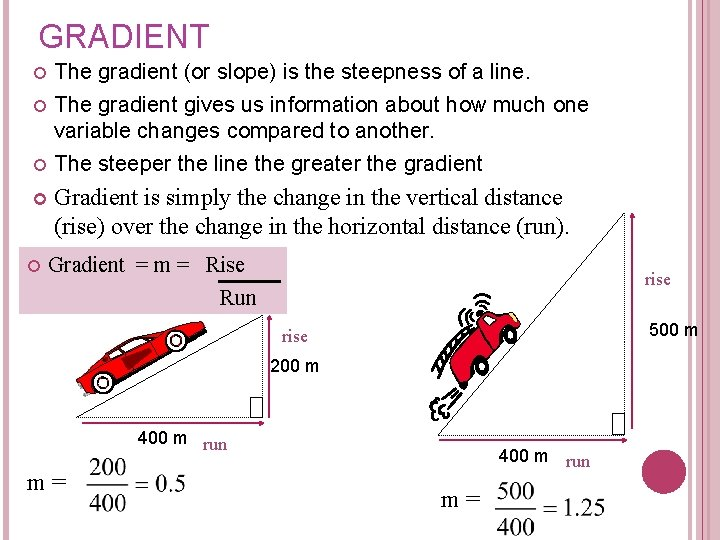GRADIENT The gradient (or slope) is the steepness of a line. The gradient gives us information about how much one variable changes compared to another. The steeper the line the greater the gradient Gradient is simply the change in the vertical distance (rise) over the change in the horizontal distance (run). Gradient = m = Rise Run rise 500 m rise 200 m 400 m run m=GRADIENT The graph of y = 2 x - 4 Gradient = m = Rise Run rise m= 8 4 = 2 8 run 4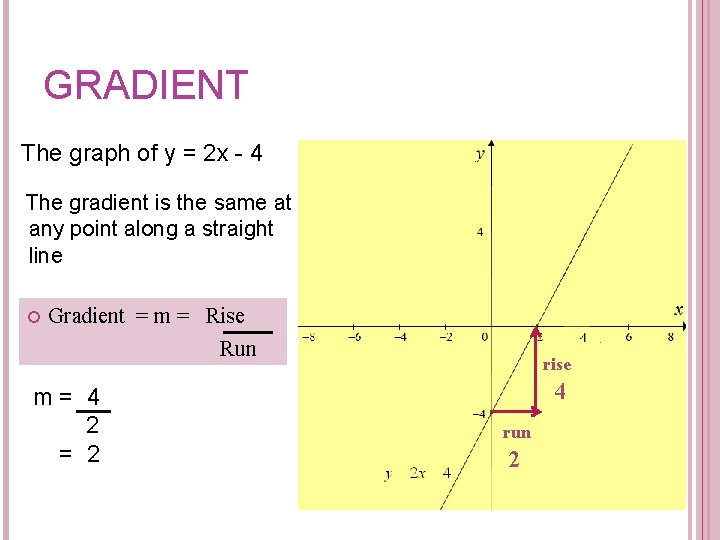GRADIENT The graph of y = 2 x - 4 The gradient is the same at any point along a straight line Gradient = m = Rise Run m= 4 2 = 2 rise 4 run 2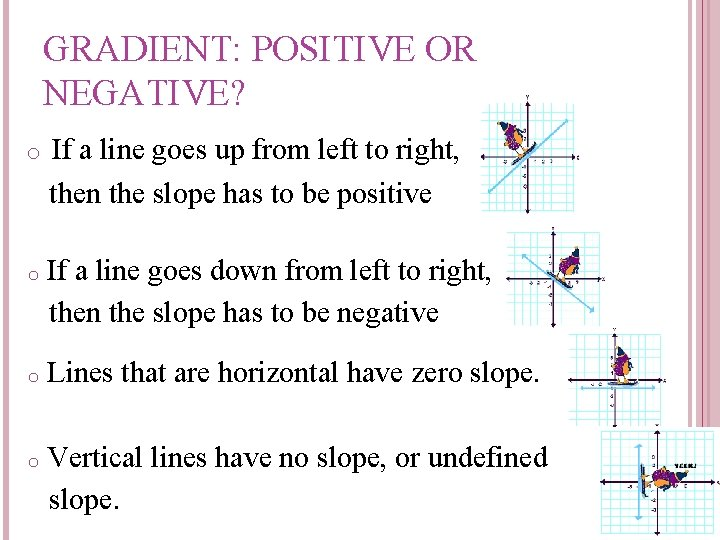GRADIENT: POSITIVE OR NEGATIVE? o If a line goes up from left to right, then the slope has to be positive o If a line goes down from left to right, then the slope has to be negative o Lines that are horizontal have zero slope. o Vertical lines have no slope, or undefined slope.GRADIENT: POSITIVE OR NEGATIVE? Positive gradient o x- value increases o y- value increases Negative gradient o x- value increases o y- value decreasesGRADIENT: DEFINITION Since the rise is simply the change in the vertical distance and the run is the change in the horizontal distance. The rise and run can be found by calculating o the change between two points on a line. o. In order to use this formula we need to know, or be able to find 2 points on the line. Eg (0, -4) & (2, 0) x 1, y 1 x 2, y 2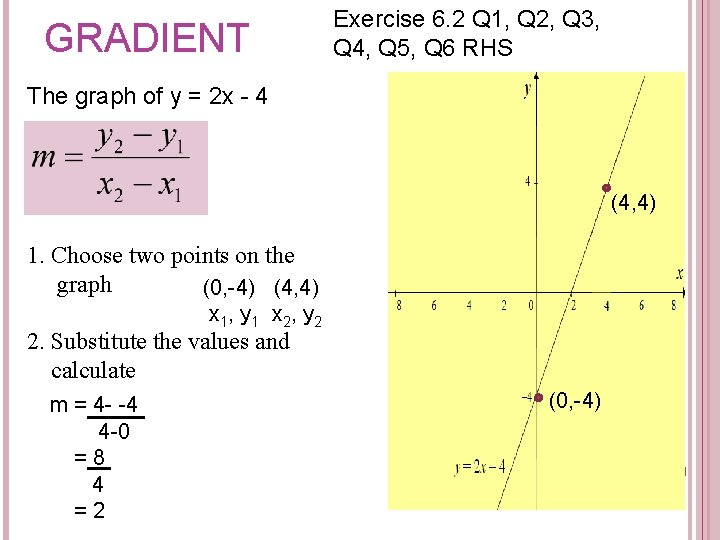GRADIENT Exercise 6. 2 Q 1, Q 2, Q 3, Q 4, Q 5, Q 6 RHS The graph of y = 2 x - 4 (4, 4) 1. Choose two points on the graph (0, -4) (4, 4) x 1, y 1 x 2, y 2 2. Substitute the values and calculate m = 4 - -4 4 -0 =8 4 =2 (0, -4)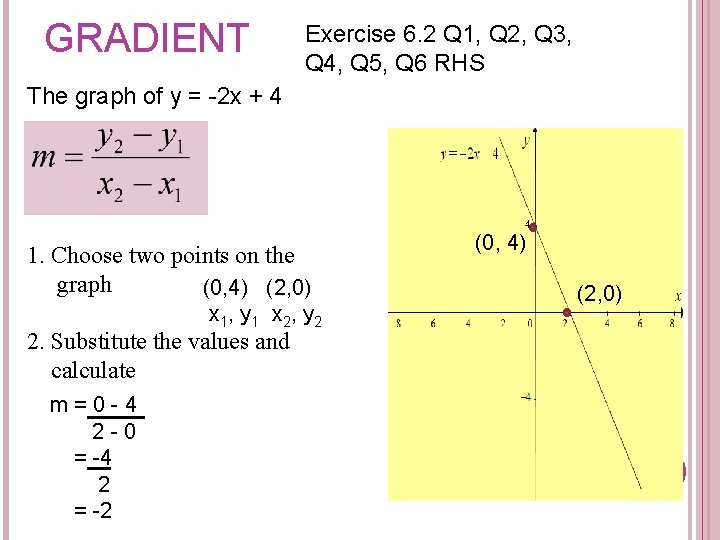GRADIENT Exercise 6. 2 Q 1, Q 2, Q 3, Q 4, Q 5, Q 6 RHS The graph of y = -2 x + 4 1. Choose two points on the graph (0, 4) (2, 0) x 1, y 1 x 2, y 2 2. Substitute the values and calculate m=0 -4 2 -0 = -4 2 = -2 (0, 4) (2, 0)INTERCEPTS The x-intercept occurs where the line cuts the x-axis. At the x- intercept the y-value always equals to 0. The y- intercept occurs where the line cuts the y-axis. At the y-intercept the x-value is always equal to 0. (2, 0) x-intercept (0, -4) y-interceptBRAIN STORM! 1. Explain how you could find the y-intercept by: a) Looking at a graph Find the coordinates for the point where the line passes through the x-axis (the xintercept), and for the point where the line passes through the y axis (the y-intercept) b) Looking at an equation Substitute y = 0 into the equation to find the x y = 2 x +3 c) Looking at X 0 Y 4 value for the x-intercept. Substitute x = 0 into the equation to find the y -value for the y-intercept. Carefully plot the points a table of values and look at the graph. OR Work out the rule used an 2 4 use the method listed 8 12 above under looking at an equation Rule: y = 2 x +4FIND THE INTERCEPTS: 1. Find the x and y intercepts of the following graphs: (2, 0) a. b. x-intercept (0, 4) y-intercept (0, -4) y-intercept c. (0, 0) y-intercept (0, 0) x-intercept d. (0, 4) y-intercept (4, 0) x-interceptFIND THE INTERCEPTS Example 1. Find the x and y intercepts of the following equation: y=3 x+2 x int: y = 0 0=3 x+2 0 -2 = 3 x +2 - 2 -2 = 3 x 3 3 X=-2 3 x – intercept = -2/3 i. e. The line cuts the x –axis at (-2/3, 0) y int: x = 0 y = 3 (0) + 2 y=0+2 y=2 y-intercept = 2 i. e. the line cuts the y axis at (0, 2)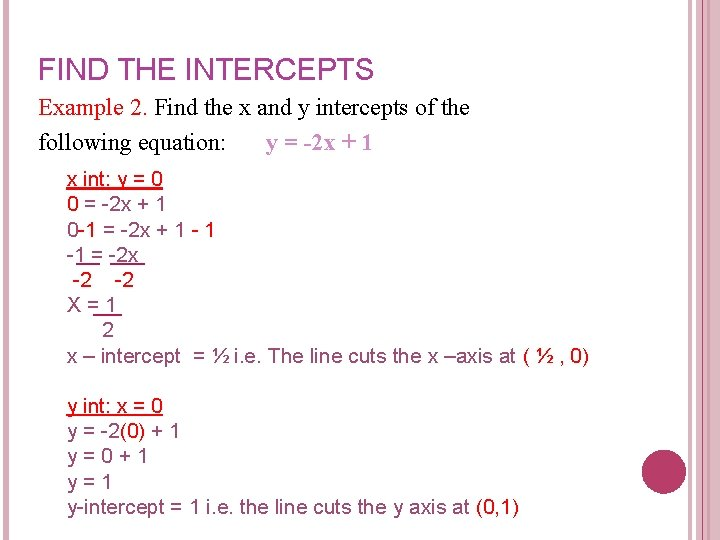FIND THE INTERCEPTS Example 2. Find the x and y intercepts of the following equation: y = -2 x + 1 x int: y = 0 0 = -2 x + 1 0 -1 = -2 x + 1 -1 = -2 x -2 -2 X=1 2 x – intercept = ½ i. e. The line cuts the x –axis at ( ½ , 0) y int: x = 0 y = -2(0) + 1 y=0+1 y=1 y-intercept = 1 i. e. the line cuts the y axis at (0, 1)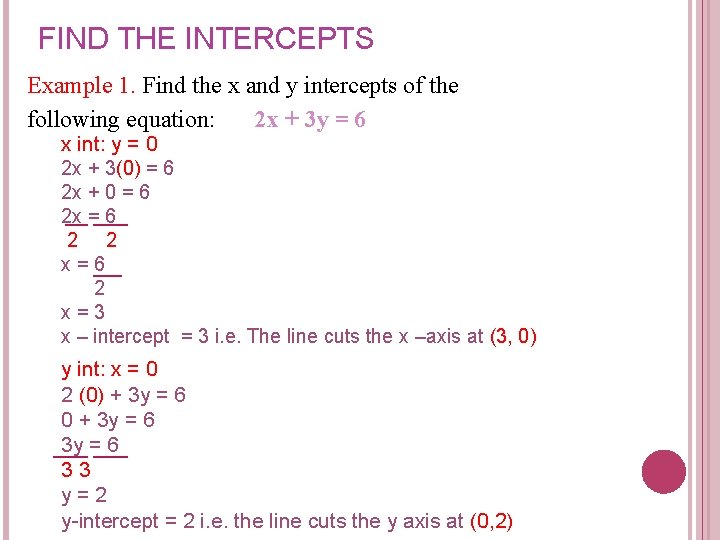FIND THE INTERCEPTS Example 1. Find the x and y intercepts of the following equation: 2 x + 3 y = 6 x int: y = 0 2 x + 3(0) = 6 2 x + 0 = 6 2 x = 6 2 2 x=6 2 x=3 x – intercept = 3 i. e. The line cuts the x –axis at (3, 0) y int: x = 0 2 (0) + 3 y = 6 0 + 3 y = 6 33 y=2 y-intercept = 2 i. e. the line cuts the y axis at (0, 2)REMEMBER!! The x-intercept occurs where the line cuts the x-axis. At the x- intercept the y-value always equals to 0. The y- intercept occurs where the line cuts the y-axis. At the y-intercept the x-value is always equal to 0. (2, 0) x-intercept (0, -4) y-intercept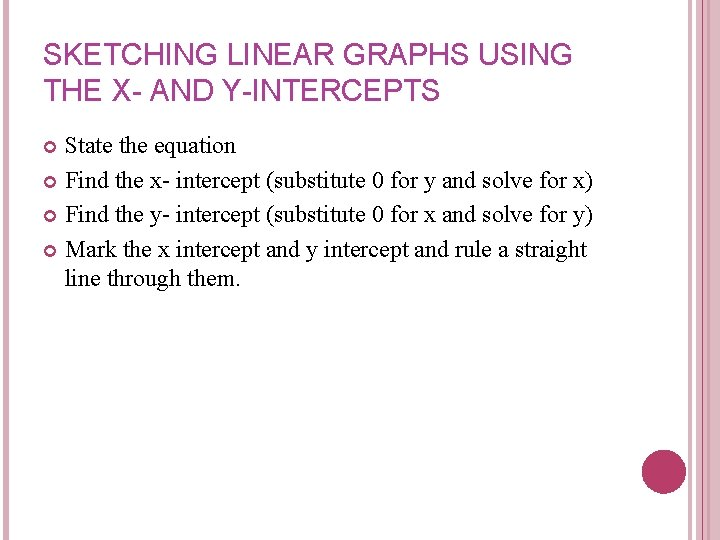SKETCHING LINEAR GRAPHS USING THE X- AND Y-INTERCEPTS State the equation Find the x- intercept (substitute 0 for y and solve for x) Find the y- intercept (substitute 0 for x and solve for y) Mark the x intercept and y intercept and rule a straight line through them.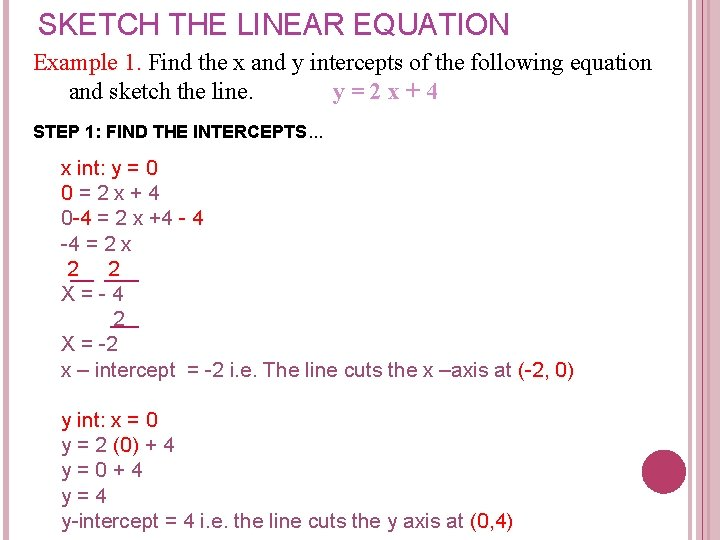SKETCH THE LINEAR EQUATION Example 1. Find the x and y intercepts of the following equation and sketch the line. y=2 x+4 STEP 1: FIND THE INTERCEPTS. . . x int: y = 0 0=2 x+4 0 -4 = 2 x +4 - 4 -4 = 2 x 2 2 X=-4 2 X = -2 x – intercept = -2 i. e. The line cuts the x –axis at (-2, 0) y int: x = 0 y = 2 (0) + 4 y=0+4 y=4 y-intercept = 4 i. e. the line cuts the y axis at (0, 4)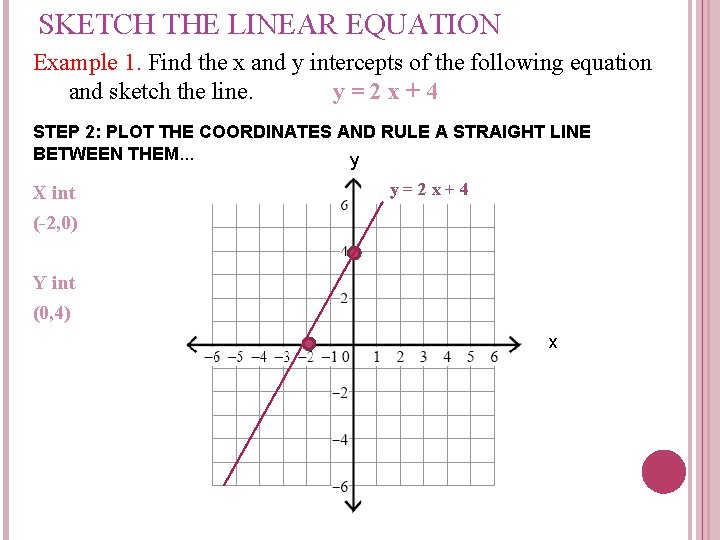SKETCH THE LINEAR EQUATION Example 1. Find the x and y intercepts of the following equation and sketch the line. y=2 x+4 STEP 2: PLOT THE COORDINATES AND RULE A STRAIGHT LINE BETWEEN THEM. . . y X int (-2, 0) y=2 x+4 Y int (0, 4) x